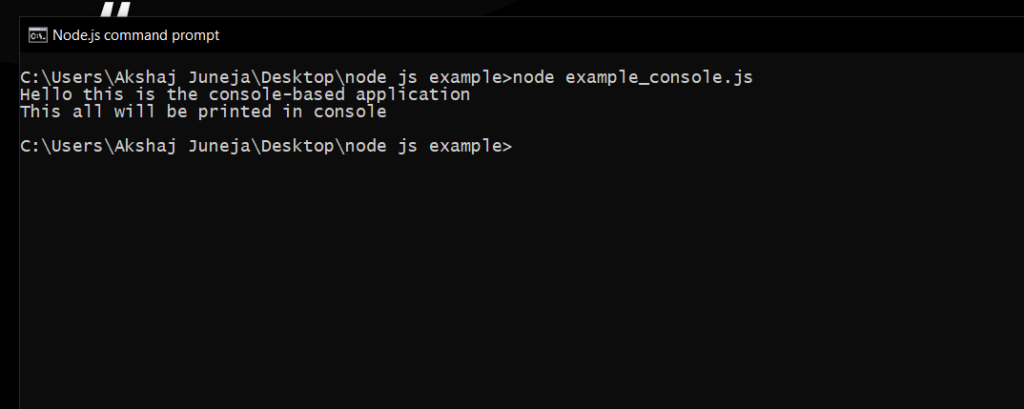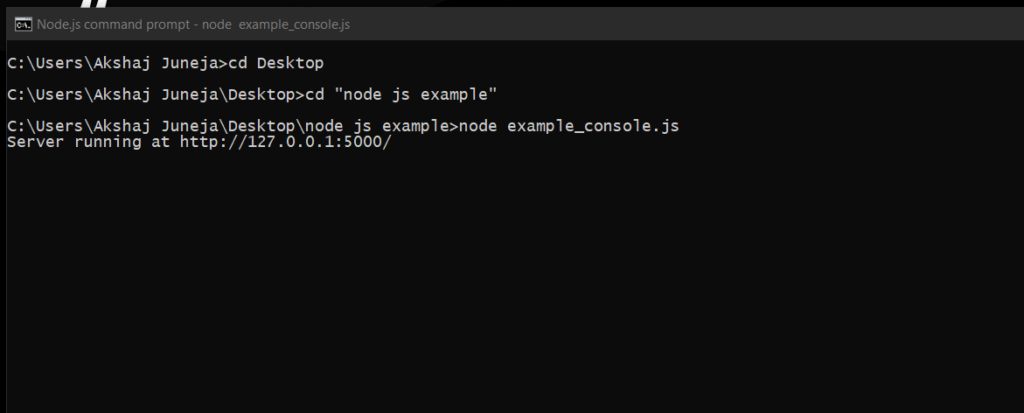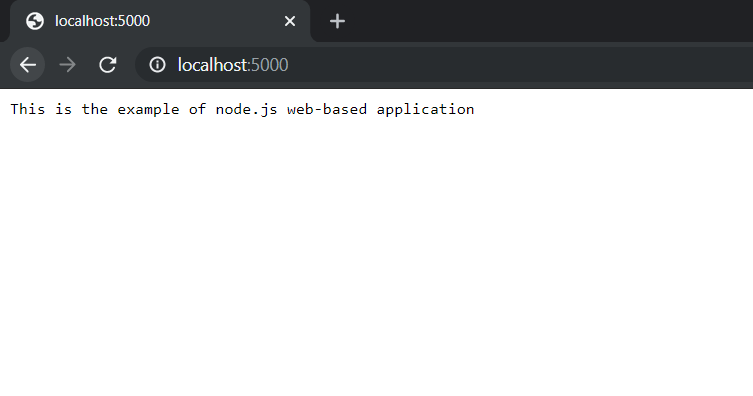Open in App
Not now

# Node.js Basics

• Difficulty Level : Medium
• Last Updated : 13 Oct, 2021

Node.js is a cross-platform JavaScript runtime environment. It allows the creation of scalable Web servers without threading and networking tools using JavaScript and a collection of “modules” that handle various core functionalities. It can make console-based and web-based node.js applications.

Datatypes: Node.js contains various types of data types similar to JavaScript.

• Boolean
• Undefined
• Null
• String
• Number

Loose Typing: Node.js supports loose typing, it means you don’t need to specify what type of information will be stored in a variable in advance. We use var keyword in Node.js to declare any type of variable. Examples are given below:

Example:

 `// Variable store number data type ` `var` `a = 35; ` `console.log(``typeof` `a); ` `  `  `// Variable store string data type ` `a = ``"GeeksforGeeks"``; ` `console.log(``typeof` `a); ` `  `  `// Variable store Boolean data type ` `a = ``true``; ` `console.log(``typeof` `a); ` `  `  `// Variable store undefined (no value) data type ` `a = undefined; ` `console.log(``typeof` `a); `

Output:

```number
string
boolean
undefined```

Objects & Functions: Node.js objects are same as JavaScript objects i.e. the objects are similar to variable and it contains many values which are written as name : value pairs. Name and value are separated by colon and every pair is separated by comma.

Example:

 `var` `company = { ` `    ``Name: ``"GeeksforGeeks"``,  ` `    ``Address: ``"Noida"``,  ` `    ``Contact: ``"+919876543210"``, ` `    ``Email: ``"abc@geeksforgeeks.org"` `}; ` ` `  `// Display the object information ` `console.log(``"Information of variable company:"``, company); ` `      `  `// Display the type of variable ` `console.log(``"Type of variable company:"``, ``typeof` `company); `

Output:

```Information of variable company: {
Name: 'GeeksforGeeks',
Contact: '+919876543210',
Email: 'abc@geeksforgeeks.org'
}
Type of variable company: object```

Functions: Node.js functions are defined using function keyword then the name of the function and parameters which are passed in the function. In Node.js, we don’t have to specify datatypes for the parameters and check the number of arguments received. Node.js functions follow every rule which is there while writing JavaScript functions.

Example:

 `function` `multiply(num1, num2) { ` ` `  `    ``// It returns the multiplication ` `    ``// of num1 and num2 ` `    ``return` `num1 * num2; ` `} ` `      `  `// Declare variable ` `var` `x = 2; ` `var` `y = 3; ` ` `  `// Display the answer returned by ` `// multiply function ` `console.log(``"Multiplication of"``, x,  ` `    ``"and"``, y, ``"is"``, multiply(x, y)); `

Output:

`Multiplication of 2 and 3 is 6`

If you observe in the above example, we have created a function called “multiply” with parameters same like JavaScript.

String and String Functions: In Node.js we can make a variable as string by assigning a value either by using single (”) or double (“”) quotes and it contains many functions to manipulate to strings.
Following is the example of defining string variables and functions in node.js.

Example:

 `var` `x = ``"Welcome to GeeksforGeeks "``; ` `  `  `var` `y = ``'Node.js Tutorials'``; ` `  `  `var` `z = [``'Geeks'``, ``'for'``, ``'Geeks'``]; ` `  `  `console.log(x); ` `  `  `console.log(y); ` `  `  `console.log(``"Concat Using (+) :"``, (x + y)); ` `  `  `console.log(``"Concat Using Function :"``, (x.concat(y))); ` `  `  `console.log(``"Split string: "``, x.split(``' '``)); ` `  `  `console.log(``"Join string: "``, z.join(``', '``)); ` `  `  `console.log(``"Char At Index 5: "``, x.charAt(5) ); `

Output:

```Welcome to GeeksforGeeks
Node.js Tutorials
Concat Using (+) : Welcome to GeeksforGeeks Node.js Tutorials
Concat Using Function : Welcome to GeeksforGeeks Node.js Tutorials
Split string:  [ 'Welcome', 'to', 'GeeksforGeeks', '' ]
Join string:  Geeks, for, Geeks
Char At Index 5:  m```

Buffer: In node.js, we have a data type called “Buffer” to store a binary data and it is useful when we are reading a data from files or receiving a packets over network.

Node.js console-based application: Make a file called console.js with the following code.

 `console.log(``'Hello this is the console-based application'``); ` ` `  `console.log(``'This all will be printed in console'``); ` ` `  `// The above two lines will be printed in the console. `

To run this file, open node.js command prompt and go to the folder where console.js file exist and write the following command. It will display content on console.The console.log() method of console class prints the message passed in the method in the console.

Node.js web-based application: Node.js web application contains different types of modules which is imported using require() directive and we have to create a server and write code for the read request and return response.
Make a file web.js with the following code.

 `// Require http module ` `var` `http = require(``"http"``);   ` ` `  `// Create server ` `http.createServer(``function` `(req, res) {   ` `  `  `    ``// Send the HTTP header    ` `    ``// HTTP Status: 200 : OK   ` `    ``// Content Type: text/plain   ` `    ``res.writeHead(200, {``'Content-Type'``: ``'text/plain'``});   ` `    `  `    ``// Send the response body as "This is the example ` `    ``// of node.js web based application"   ` `   ``res.end(``'This is the example of node.js web-based application \n'``);   ` ` `  `// Console will display the message   ` `}).listen(5000,  ` `    ``()=>console.log(``'Server running at http://127.0.0.1:5000/'``)); `

To run this file follow the steps as given below:

• Search the node.js command prompt in the search bar and open the node.js command prompt.
• Go to the folder using cd command in command prompt and write the following command node web.js• Now the server has started and go to the browser and open this url localhost:5000You will see the response which you have sent back from web.js in the browser. If any changes are made in the web.js file then again run the command node web.js and refresh the tab in the browser.

My Personal Notes arrow_drop_up
Related Articles